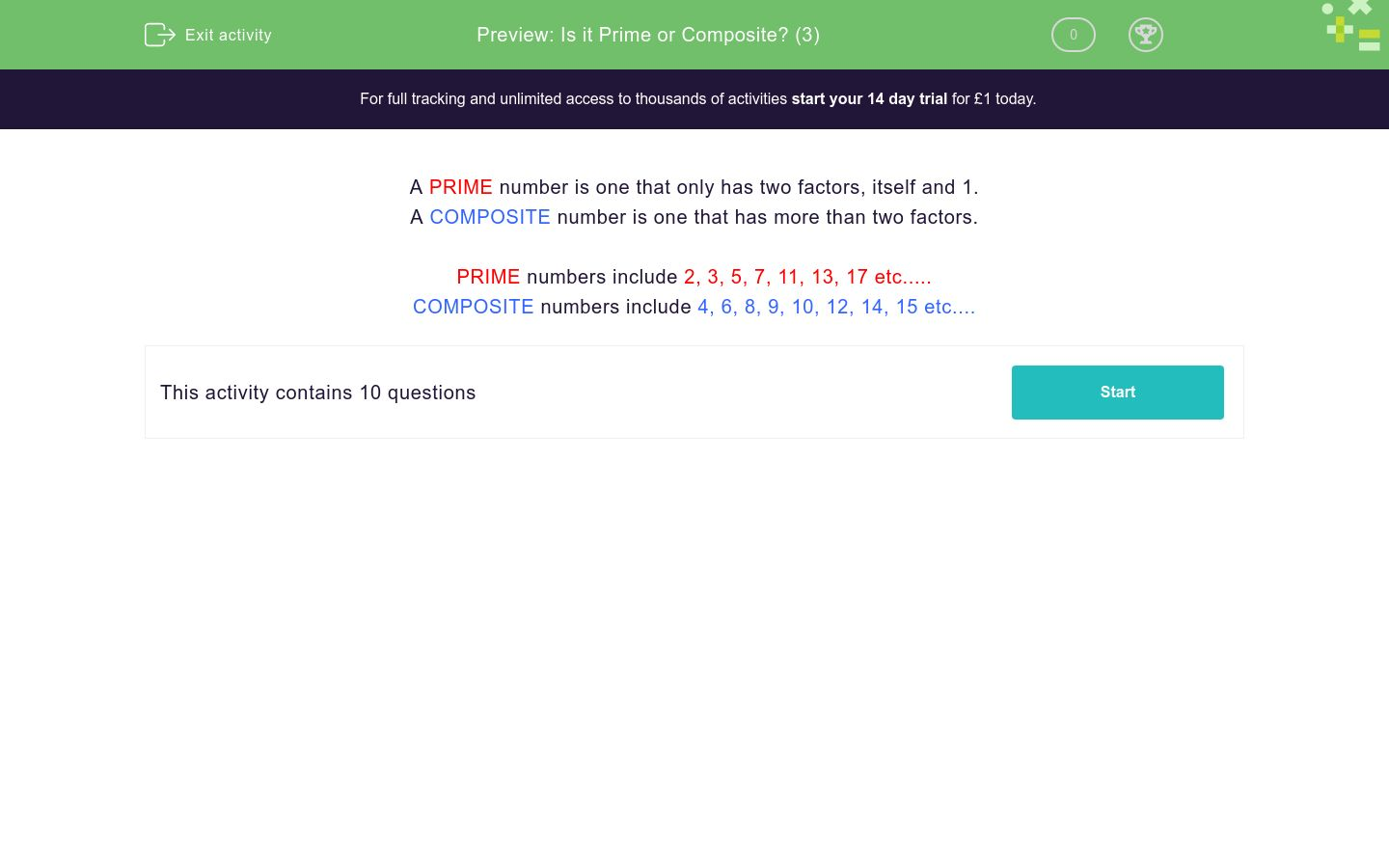# Is it Prime or Composite? (3)

In this worksheet, students decide whether or not a given number is prime or composite.Key stage:  KS 3

Curriculum topic:  Number

Curriculum subtopic:  Use Concepts and Vocabulary for All Numbers

Difficulty level:### QUESTION 1 of 10

A PRIME number is one that only has two factors, itself and 1.

A COMPOSITE number is one that has more than two factors.

PRIME numbers include 2, 3, 5, 7, 11, 13, 17 etc.....

COMPOSITE numbers include 4, 6, 8, 9, 10, 12, 14, 15 etc....

Is the following number prime or composite?

95

Composite

Prime

Is the following number prime or composite?

91

Composite

Prime

Is the following number prime or composite?

97

Composite

Prime

Is the following number prime or composite?

117

Composite

Prime

Is the following number prime or composite?

119

Composite

Prime

Is the following number prime or composite?

127

Composite

Prime

Is the following number prime or composite?

131

Composite

Prime

Is the following number prime or composite?

141

Composite

Prime

Is the following number prime or composite?

233

Composite

Prime

Is the following number prime or composite?

231

Composite

Prime

• Question 1

Is the following number prime or composite?

95

Composite
• Question 2

Is the following number prime or composite?

91

Composite
• Question 3

Is the following number prime or composite?

97

Prime
• Question 4

Is the following number prime or composite?

117

Composite
• Question 5

Is the following number prime or composite?

119

Composite
• Question 6

Is the following number prime or composite?

127

Prime
• Question 7

Is the following number prime or composite?

131

Prime
• Question 8

Is the following number prime or composite?

141

Composite
• Question 9

Is the following number prime or composite?

233

Prime
• Question 10

Is the following number prime or composite?

231

Composite
---- OR ----

Sign up for a £1 trial so you can track and measure your child's progress on this activity.

### What is EdPlace?

We're your National Curriculum aligned online education content provider helping each child succeed in English, maths and science from year 1 to GCSE. With an EdPlace account you’ll be able to track and measure progress, helping each child achieve their best. We build confidence and attainment by personalising each child’s learning at a level that suits them.

Get started#### Similar activities you may like

Start your £1 trial today.
Subscribe from £10/month.